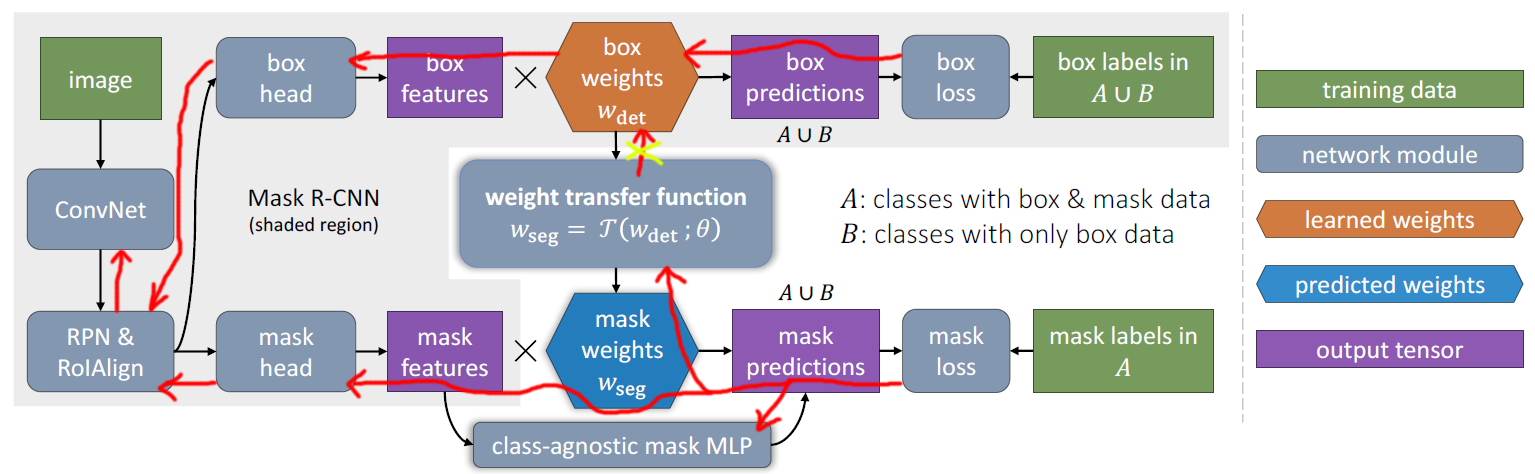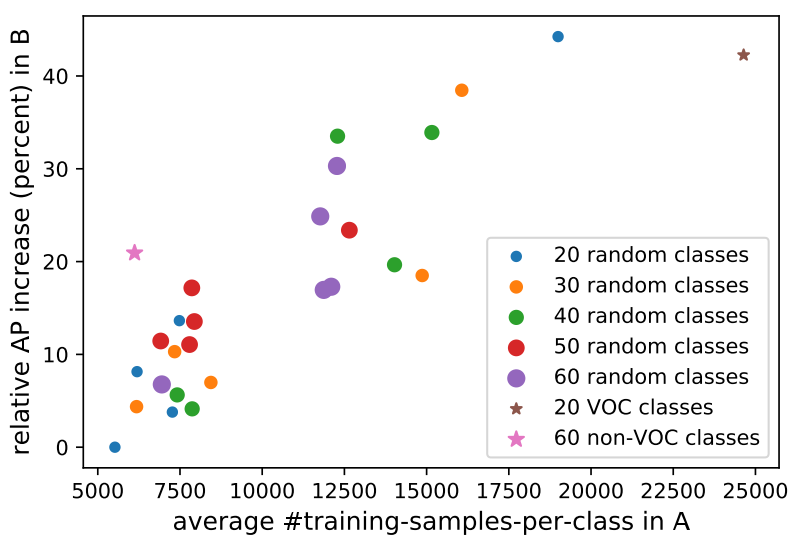Jarvis' Blog (总有美丽的风景让人流连) 总有美丽的风景让人流连

2017-12-14
Jarvis
Post

## 1. Introduction

1. 给定一个多类别的数据集, 一个有实例分割标注的小型子集, 其他样本仅有 bounding box 标注.
2. 训练一个网络利用 1 中的数据来分割所有的样本.

• Training: 使用所有的 Bbox 训练整个网络参数, 使用分割的标注数据训练加权迁移函数
• Inference: 应用加权迁移函数到 Bbox 回归的网络参数上作为实例分割的网络参数.

• COCO 数据集 - 80类: 分为有分割标注和无分割标注两部分
• Visual Genome (VG) 数据集 - 3000类: 该数据集质量不高, 且是语义分割的标注, 所以由于物体语义上的重叠导致评估结果不可靠.

## 2. Learning to Segment Every Thing

• $$w^c_{det}$$ 是目标检测的权重, 特指 bbox 子网络中的最后一层卷积的参数;
• $$w^c_{seg}$$ 是目标分割的权重, 特指 mask 子网络中的最后一层卷积的参数.

$w^c_{seg} = \mathcal{T}(w^c_{det};\theta),$### 2.2 训练细节

• A: 包含实例分割标注 (那么相应的 bbox 也可以从分割图中很容易的获取)
• B: 仅包含 bbox 标注

#### 多阶段训练

• 第一阶段: 仅使用 $$A\cup B$$ 中的 bbox 标注训练 bbox 分支
• 第二阶段: 固定主干和 bbox 分支的权重不变, 使用 A 训练 mask 分支.

### 2.3 混合 FCN+MLP 的 mask 头部

• FCN, 使用全卷积网络产生 M×M 的 mask

1. mask features × mask weights, 该预测是类别相关的, 所以会产生 $$K\times M\times M$$ 的输出, $$K$$ 是类别数.
2. mask MLP , 该预测是类别无关的, 所以会产生 $$1\times M\times M$$ 的输出,

### 2.4 Baseline 和 oracle

• oracle 是 Mask R-CNN 在数据集 $$A\cup B$$ 上使用全部的分割标注来训练的 Mask R-CNN, 容易看出 oracle 是全监督的, 从而也是本文提出的部分监督的一个上界.

## 3. 对照实验

2. 预训练的 GloVe 向量
3. Mask R-CNN 的 box 分支的分类权重 cls
4. Mask R-CNN 的 box 分支的回归权重 box
5. cls + box (concatenate)
cls + box

2. 2-fc-layer + ReLU
3. 2-fc-layer + LeakyReLU
4. 3-fc-layer + ReLU
5. 3-fc-layer + LeakyReLU
2-fc-layer + LeakyReLU
MLP 分支的影响 1. baseline
2. baseline + MLP
3. transfer
4. transfer + MLP

2. transfer + stage-wise
3. baseline + end2end
4. transfer + end2end
5. transfer + end2end + stop gradient on $$w_{det}$$

2. 30 ~ 50
3. ……
4. 60 ~ 20
5. 20 VOC ~ 60 non-VOC
6. 60 non-VOC ~ 20 VOCContent